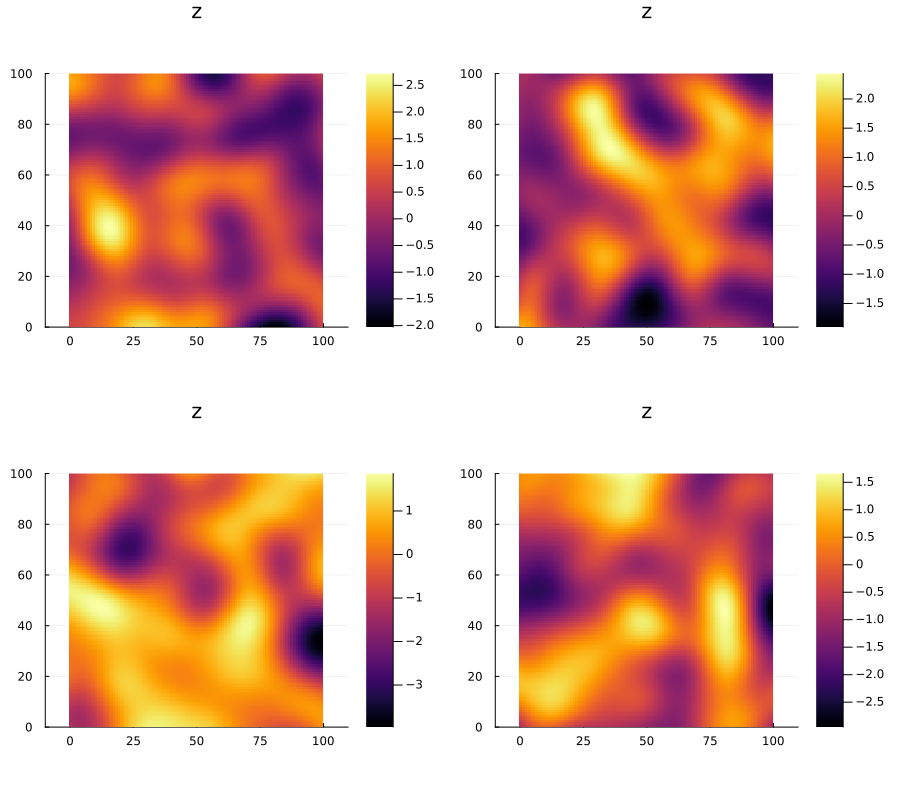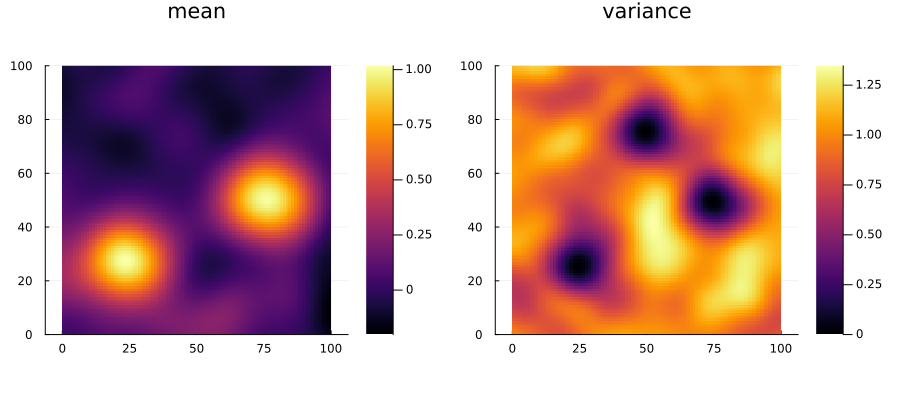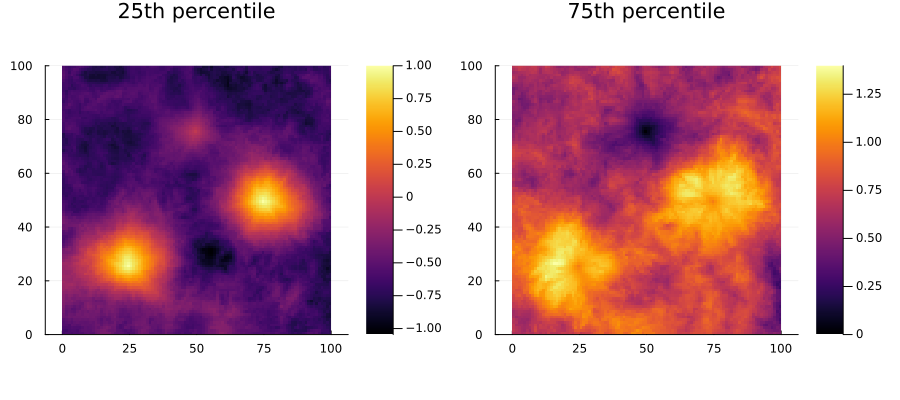# Ensembles

GeoStatsBase.EnsembleType
Ensemble

An ensemble of geostatistical realizations.

The result of a geostatistical simulation solver is a set of geospatial data (a.k.a. realizations). This set can be interpreted as an empirical approximation of the random field of interest.

Notes

Ensemble objects are typically created by a call to the solve function on a SimulationProblem.

Consider the following solution to a conditional simulation problem:

using GeoStats
using Plots, GeoStatsPlots

# geospatial samples
S = let
coord = [(25.,25.), (50.,75.), (75.,50.)]
table = (z=[1.,0.,1.],)
georef(table, coord)
end

# simulation domain
D = CartesianGrid(100, 100)

# request 100 realizations
problem = SimulationProblem(S, D, :z, 100)

# LU Gaussian simulation
solver = LUGS(:z => (variogram=GaussianVariogram(range=30.),))

# realizations form an ensemble
ensemble = solve(problem, solver)
2D Ensemble
domain:    100×100 CartesianGrid{2,Float64}
variables: z (Float64)
N° reals:  100

We can visualize a few realizations in the ensemble:

z1 = ensemble
z2 = ensemble
z3 = ensemble
z4 = ensemble

plot(plot(z1), plot(z2),
plot(z3), plot(z4),
size=(900,800))or alternatively, the mean and variance:

m = mean(ensemble)
v = var(ensemble)

p1 = plot(m, title="mean")
p2 = plot(v, title="variance")

plot(p1, p2)or the 25th and 75th percentiles:

a = quantile(ensemble, 0.25)
b = quantile(ensemble, 0.75)

p1 = plot(a, title="25th percentile")
p2 = plot(b, title="75th percentile")

plot(p1, p2)All these objects are examples of geospatial data as described in the Data section.